Advertisement Remove all ads

# Balbharati solutions for Chemistry 12th Standard HSC for Maharashtra State Board chapter 10 - Halogen Derivatives [Latest edition]

Advertisement Remove all ads

#### Chapters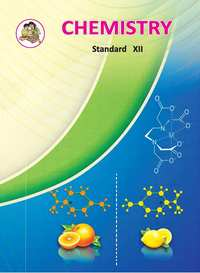## Chapter 10: Halogen Derivatives

Exercise
Exercise [Pages 231 - 233]

### Balbharati solutions for Chemistry 12th Standard HSC for Maharashtra State Board Chapter 10 Halogen Derivatives Exercise [Pages 231 - 233]

Exercise | Q 1.01 | Page 231

Choose the most correct option.

The correct order of increasing reactivity of C-X bond towards nucleophile in the following compounds is

(I)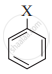(II)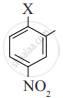(III) (CH3)3 C-X

(IV) (CH3)2 CH-X

• I < II < III < IV

• II < I < III < IV

• III < IV < II < I

• IV < III < I < II

Exercise | Q 1.02 | Page 231

Choose the most correct option.

$\ce{CH3 - CH = CH2 ->[HI][Peroxide]}$

The major product of the above reaction is, _______.

• I - CH2 - CH = CH2

• CH3 - CH2 - CH2I

• $\begin{array}{cc} \ce{CH3-CH-CH3}\\ |\\ \ce{I}\end{array}$

• $\begin{array}{cc} \ce{CH3-CH-CH2}\\ \phantom{.....}|\phantom{.....}|\\ \phantom{.......}\ce{I}\phantom{....}\ce{OH} \end{array}$

Exercise | Q 1.03 | Page 231

Choose the most correct option.

Which of the following is likely to undergo racemization during alkaline hydrolysis?

(I)

$\begin{array}{cc} \ce{CH3-CH-C2H5}\\ |\\ \ce{Cl}\end{array}$

(II)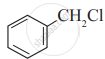(III)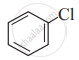(IV)

$\begin{array}{cc} \ce{\phantom{.......}CH3}\\ \phantom{.....}|\\ \ce{CH3-CH}\\ \phantom{.....}|\\ \ce{\phantom{..........}CH2Cl} \end{array}$

• Only I

• Only II

• II and IV

• Only IV

Exercise | Q 1.04 | Page 231

Choose the most correct option.

The best method for the preparation of alkyl fluorides is _______.

• Finkelstein reaction

• Swartz reaction

• Free radical fluorination

• Sandmeyer's reaction

Exercise | Q 1.05 | Page 231

Choose the most correct option.

Identify the chiral molecule from the following.

a. 1 - Bromobutane

b. 1,1-Dibromobutane

c. 2,3-Dibromobutane

d. 2-Bromobutane

Exercise | Q 1.06 | Page 231

Choose the most correct option.

An alkyl chloride on Wurtz reaction gives 2,2,5,5-tetramethylhexane. The same alkyl chloride on reduction with a zinc-copper couple in alcohol gives hydrocarbon with molecular formula C5H12. What is the structure of alkyl chloride?

• $\begin{array}{cc} \ce{CH3}\\ |\phantom{...}\\ \ce{CH3-C-CH2Cl}\\ |\phantom{...}\\ \ce{CH3} \end{array}$

• $\begin{array}{cc} \ce{CH3\phantom{..}}\\ |\phantom{.....}\\ \ce{CH3-C-CH2CH3}\\ |\phantom{.....}\\ \ce{Cl\phantom{....}} \end{array}$

• $\begin{array}{cc} \ce{CH3-CH2-CH-Cl}\\ \phantom{......}|\\ \ce{\phantom{.........}CH3} \end{array}$

• $\begin{array}{cc} \ce{CH3-CH-CH-CHCl}\\ |\phantom{.....}|\phantom{...}\\ \ce{\phantom{..}CH3} \ce{\phantom{..}CH3\phantom{..}} \end{array}$

Exercise | Q 1.07 | Page 231

Choose the most correct option.

Butanenitrile may be prepared by heating _______.

• propanol with KCN

• butanol with KCN

• n-butyl chloride with KCN

• n-propyl chloride with KCN

Exercise | Q 1.08 | Page 232

Choose the most correct option.

Choose the compound from the following that will react fastest by SN1 mechanism

• 1-Iodobutane

• 1-Iodopropane

• 2-Iodo-2 methylbutane

• 2-Iodo-3-methylbutane

Exercise | Q 1.09 | Page 232

Choose the most correct option.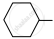$\ce{Cl + Mg ->[dry][ether] A ->[H2O]}$

The product ‘B’ in the above reaction sequence is, _______.

•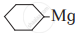•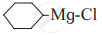•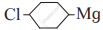•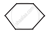Exercise | Q 1.1 | Page 232

Choose the most correct option.

Which of the following is used as a source of dichlorocarbene?

• tetrachloromethane

• chloroform

• iodoform

• DDT

Exercise | Q 2.1 | Page 232

Write IUPAC name of the following compound.

$\begin{array}{cc} \ce{CH3 - CH = C - CH - Br}\\ \phantom{......}|\phantom{....}|\\ \ce{\phantom{......}H3C} \ce{\phantom{...}CH3} \end{array}$

Exercise | Q 2.1 | Page 232

Write IUPAC name of the following compound.

$\begin{array}{cc} \ce{CH3 - CH - CH - CH2 - CH3}\\ \phantom{......}|\phantom{....}|\\ \ce{\phantom{........}Cl} \ce{\phantom{..}CH3} \end{array}$

Exercise | Q 2.1 | Page 232

Write IUPAC name of the following compound.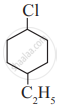Exercise | Q 2.1 | Page 232

Write IUPAC name of the following compound.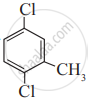Exercise | Q 2.2 | Page 232

Write the structure and IUPAC name of the major product in the following reaction.

$\begin{array}{cc} \ce{CH3 - CH - CH2Cl + NaI ->[Acetone]}\\ |\phantom{..................}\\ \ce{CH3\phantom{...............}} \end{array}$

Exercise | Q 2.2 | Page 232

Write the structure and IUPAC name of the major product in the following reaction.

CH3 - CH2Br + SbF3

Exercise | Q 2.2 | Page 232

Write the structure and IUPAC name of the major product in the following reaction.

$\begin{array}{cc} \ce{CH3 - CH - CH = CH2 + HBr ->[peroxide]}\\ |\phantom{........................}\\ \ce{CH3\phantom{.....................}} \end{array}$

Exercise | Q 2.2 | Page 232

Write the structure and IUPAC name of the major product in the following reaction.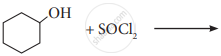Exercise | Q 2.2 | Page 232

Write the structure and IUPAC name of the major product in the following reaction.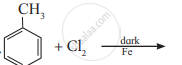Exercise | Q 2.3 | Page 232

Identify chiral molecule/s from the following.

a.

$\begin{array}{cc}\ce{CH3 - CH - CH2 - CH3}\\|\phantom{.........}\\\ce{OH\phantom{.......}}\end{array}$

b.

$\begin{array}{cc}\ce{CH3 - CH2 - CH - CH2 - CH3}\\ |\\\ce{Br}\end{array}$

c. CH3 - CH - CH2 - CH2Br

d.

$\begin{array}{cc}\ce{CH3 - CH - CH2 - CH3}\\ |\phantom{.........}\\\ce{CH3\phantom{......}}\end{array}$

Exercise | Q 2.4 | Page 232

from the following pair would undergo SN2 faster from the other?

a.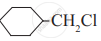b.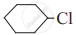Exercise | Q 2.4 | Page 232

from the following pair would undergo SN2 faster from the other?

a. CH3CH2CH2I      b. CH3CH2CH2Cl

Exercise | Q 2.5 | Page 232

Complete the following reaction giving major products.

$\ce{CH3 - CH = CH2 ->[HBr][peroxide] A ->[alc. KOH] B}$

Exercise | Q 2.5 | Page 232

Complete the following reaction giving major products.

$\begin{array}{cc}\ce{CH3 - CH = CH2 ->[Red P/Br2] A ->[Ag2O/H2O]B}\\|\phantom{.........................}\\ \ce{OH\phantom{.......................}}\end{array}$

Exercise | Q 2.5 | Page 232

Complete the following reaction giving major products.

$\begin{array}{cc}\ce{CH3\phantom{.....}}\\|\phantom{.......}\\\ce{CH3 - C - CH2 - Cl ->[Na/dry ether]}\\|\phantom{.......}\\ \ce{CH3\phantom{.....}}\end{array}$

Exercise | Q 2.5 | Page 232

Complete the following reaction giving major products.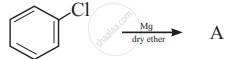Exercise | Q 2.6 | Page 233

Name the reagent used to bring about the following conversion.

Bromoethane to ethoxyethane

Exercise | Q 2.6 | Page 233

Name the reagent used to bring about the following conversion.

1-Chloropropane to 1-nitropropane

Exercise | Q 2.6 | Page 233

Name the reagent used to bring about the following conversion.

Ethyl bromide to ethyl isocyanide

Exercise | Q 2.6 | Page 233

Name the reagent used to bring about the following conversion.

Chlorobenzene to biphenyl

Exercise | Q 2.7 | Page 233

Arrange the following in the increasing order of boiling points.

1. 1-Bromopropane
2. 2- Bromopropane
3. 1- Bromobutane
4. 1-Bromo-2-methylpropane
Exercise | Q 2.8 | Page 233

Match the pairs.

 Column I Column II $\begin{array}{cc}\ce{CH3CH - CH3}\\|\phantom{....}\\\ce{X\phantom{....}}\end{array}$ vinyl halide CH2 = CH - CH2X alkyl halide CH2 = CH - X allyl halide benzyl halide aryl halide
Exercise | Q 3.1 | Page 233

Give reasons:

Haloarenes are less reactive than haloalkanes.

Exercise | Q 3.2 | Page 233

Give reason:

Alkyl halides though polar are immiscible with water.

Exercise | Q 3.3 | Page 233

Give reason:

Reactions involving Grignard reagent must be carried out under anhydrous condition.

Exercise | Q 3.4 | Page 233

Give reason:

Alkyl halides are generally not prepared by free radical halogenation of alkanes.

Exercise | Q 4 | Page 233

Distinguish between SN1 and SN2 mechanism of substitution reaction.

Exercise | Q 5 | Page 233

Explain - Optical isomerism in 2-chlorobutane.

Exercise | Q 6.1 | Page 233

Convert the following:

Propene to propan-1-ol

Exercise | Q 6.2 | Page 233

Convert the following:

Benzyl alcohol to benzyl cyanide

Exercise | Q 6.3 | Page 233

Convert the following:

Ethanol to propane nitrile

Exercise | Q 6.4 | Page 233

Convert the following:

But-1-ene to n-butyl iodide

Exercise | Q 6.5 | Page 233

Convert the following:

2-Chloropropane to propan-1-ol

Exercise | Q 6.6 | Page 233

Convert the following:

tert-Butyl bromide to isobutyl bromide

Exercise | Q 6.7 | Page 233

How the following conversions can be carried out?

Aniline to chlorobenzene

Exercise | Q 6.8 | Page 233

How will you bring about the following conversions?

Propene to 1-nitropropane

Exercise | Q 7.1 | Page 233

HCl is added to a hydrocarbon ‘A’ (C4H8) to give a compound ‘B’ which on hydrolysis with aqueous alkali forms tertiary alcohol ‘C’ (C4H10O). Identify ‘A’ ,‘B’ and ‘C’.

Exercise | Q 7.2 | Page 233

Complete the following reaction sequence by writing the structural formulae of the organic compound 'A', 'B' and 'C'.

$\ce{2-Bromobutane->[alc.KOH]A->[][Br2]B->[][NaNH2]C}$

Exercise | Q 7.2 | Page 233

Complete the following reaction sequence by writing the structural formulae of the organic compound 'A', 'B' and 'C'.

$\ce{Isopropyl alcohol ->[\triangle][PBr3] A ->[][NH3 excess] B}$

Exercise | Q 7.3 | Page 233

Observe the following and answer the question given below.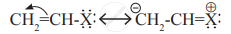Name the type of halogen derivative.

Exercise | Q 7.3 | Page 233

Observe the following and answer the question given below.Comment on the bond length of C–X bond in it.

Exercise | Q 7.3 | Page 233

Observe the following and answer the question given below.Can it react by SN1 mechanism? Justify your answer.

Advertisement Remove all ads

## Chapter 10: Halogen Derivatives

Exercise## Balbharati solutions for Chemistry 12th Standard HSC for Maharashtra State Board chapter 10 - Halogen Derivatives

Balbharati solutions for Chemistry 12th Standard HSC for Maharashtra State Board chapter 10 (Halogen Derivatives) include all questions with solution and detail explanation. This will clear students doubts about any question and improve application skills while preparing for board exams. The detailed, step-by-step solutions will help you understand the concepts better and clear your confusions, if any. Shaalaa.com has the Maharashtra State Board Chemistry 12th Standard HSC for Maharashtra State Board solutions in a manner that help students grasp basic concepts better and faster.

Further, we at Shaalaa.com provide such solutions so that students can prepare for written exams. Balbharati textbook solutions can be a core help for self-study and acts as a perfect self-help guidance for students.

Concepts covered in Chemistry 12th Standard HSC for Maharashtra State Board chapter 10 Halogen Derivatives are Classification of Halogen Derivatives, Nomenclature of Halogen Derivatives, Methods of Preparation of Alkyl Halides, Physical Properties, Optical Isomerism in Halogen Derivatives, Chemical Properties, Reaction with Active Metals, Uses and Environmental Effects of Some Polyhalogen Compounds, Nomenclature, Reactions of Haloalkanes - Elimination Reactions.

Using Balbharati 12th Board Exam solutions Halogen Derivatives exercise by students are an easy way to prepare for the exams, as they involve solutions arranged chapter-wise also page wise. The questions involved in Balbharati Solutions are important questions that can be asked in the final exam. Maximum students of Maharashtra State Board 12th Board Exam prefer Balbharati Textbook Solutions to score more in exam.

Get the free view of chapter 10 Halogen Derivatives 12th Board Exam extra questions for Chemistry 12th Standard HSC for Maharashtra State Board and can use Shaalaa.com to keep it handy for your exam preparation

Advertisement Remove all ads
Share
Notifications

View all notifications

Forgot password?